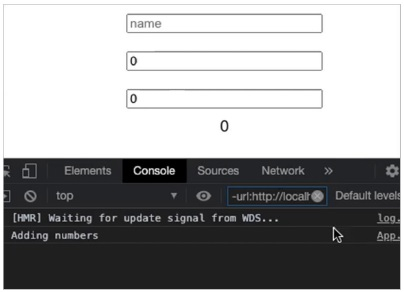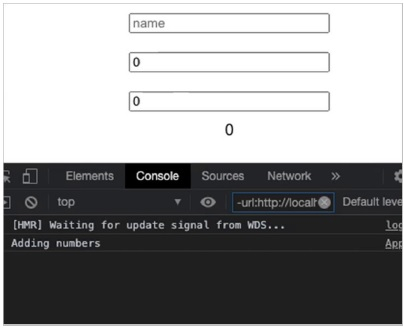# ReactJS – useCallback hook

In this article, we are going to see how to optimize a React application by passing a memoized function.

This hook is used to optimize a React application by returning a memoized function which helps to prevent unnecessary re-rendering of a function. This hook stores the cached value of the function and only updates the function if the passed dependencies changes.

## Syntax

const memoizedCallback = useCallback(() => {doSomething(a, b); }, [a, b],);

Here, doSomething() function will only be called again on the next re-render if the values of a or b changes; otherwise only the cached version of the function is passed.

Note: useCallback(fn, []) is the same as useMemo(() => fn, []).

## Without useCallback Hook

### Example

In this example, we will build a React application which has 3 input fields and it will display the sum of two numbers returned by the function add.

App.jsx

import React, { useState } from 'react';

const App = () => {
const [name, setName] = useState('');
const [num1, setNum1] = useState(0);
const [num2, setNum2] = useState(0);
return (
<div>
<input
placeholder="name"
value={name}
onChange={(e) => setName(e.target.value)}
/>
<input
placeholder="Value 1"
value={num1}
onChange={(e) => setNum1(e.target.value)}
/>
<input
placeholder="Value 2"
value={num2}
onChange={(e) => setNum2(e.target.value)}
/>
{ans}
</div>
);
};

const adder = (a1, a2) => {
return parseInt(a1) + parseInt(a2);
};

export default App;

In the above example, when the user types the name, even then the add function is called on every re-render which makes the React application not optimized. Add function is getting called on every re-render as when the user types, the state changes which re-renders the app.

### Output

This will produce the following result.## With useCallback Hook

### Example

App.jsx

import React, { useState,useCallback } from 'react';

const App = () => {
const [name, setName] = useState('');
const [num1, setNum1] = useState(0);
const [num2, setNum2] = useState(0);
const ans = useCallback(() => {
}, [val1, val2]);
return (
<div>
<input
placeholder="name"
value={name}
onChange={(e) => setName(e.target.value)}
/>
<input
placeholder="Value 1"
value={num1}
onChange={(e) => setNum1(e.target.value)}
/>
<input
placeholder="Value 2"
value={num2}
onChange={(e) => setNum2(e.target.value)}
/>
{ans}
</div>
);
};
const adder = (a1, a2) => {
export default App;